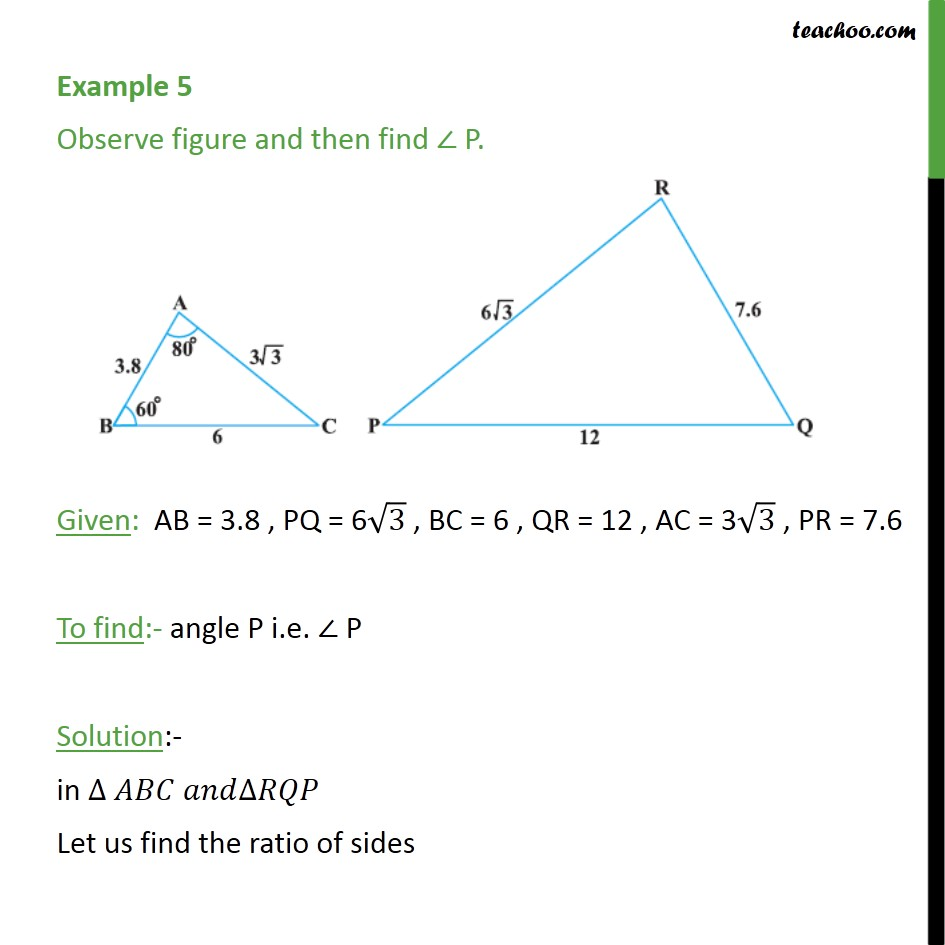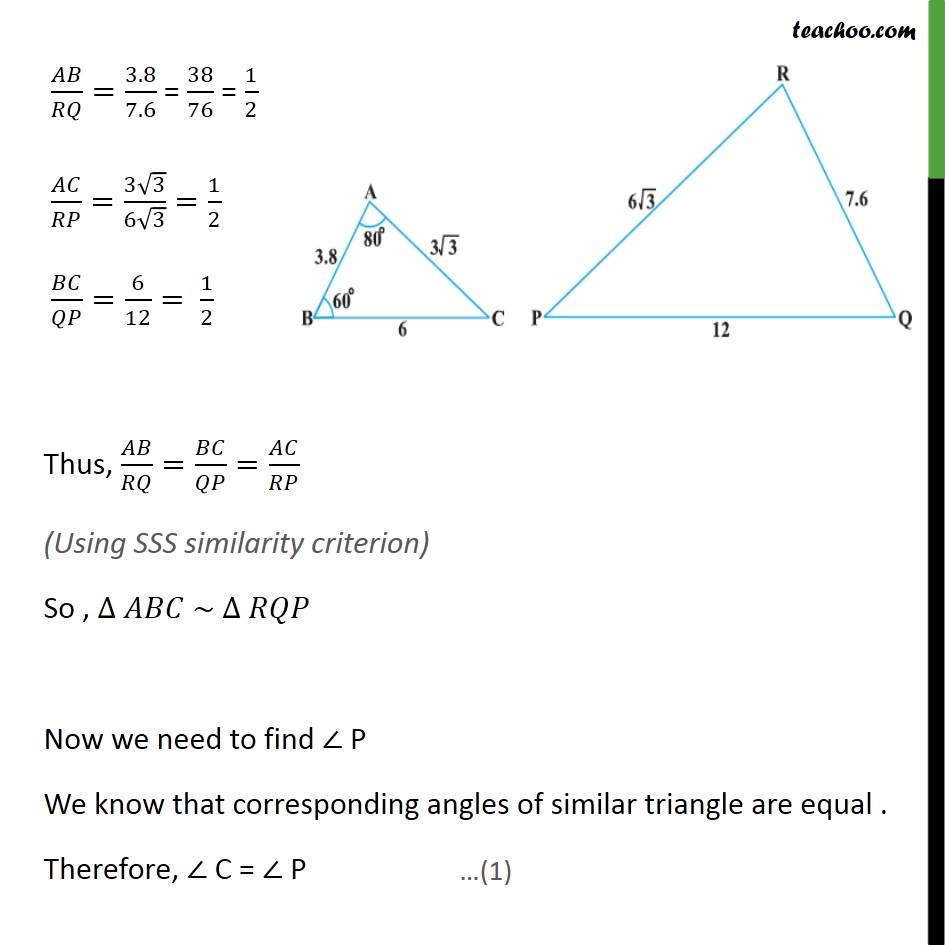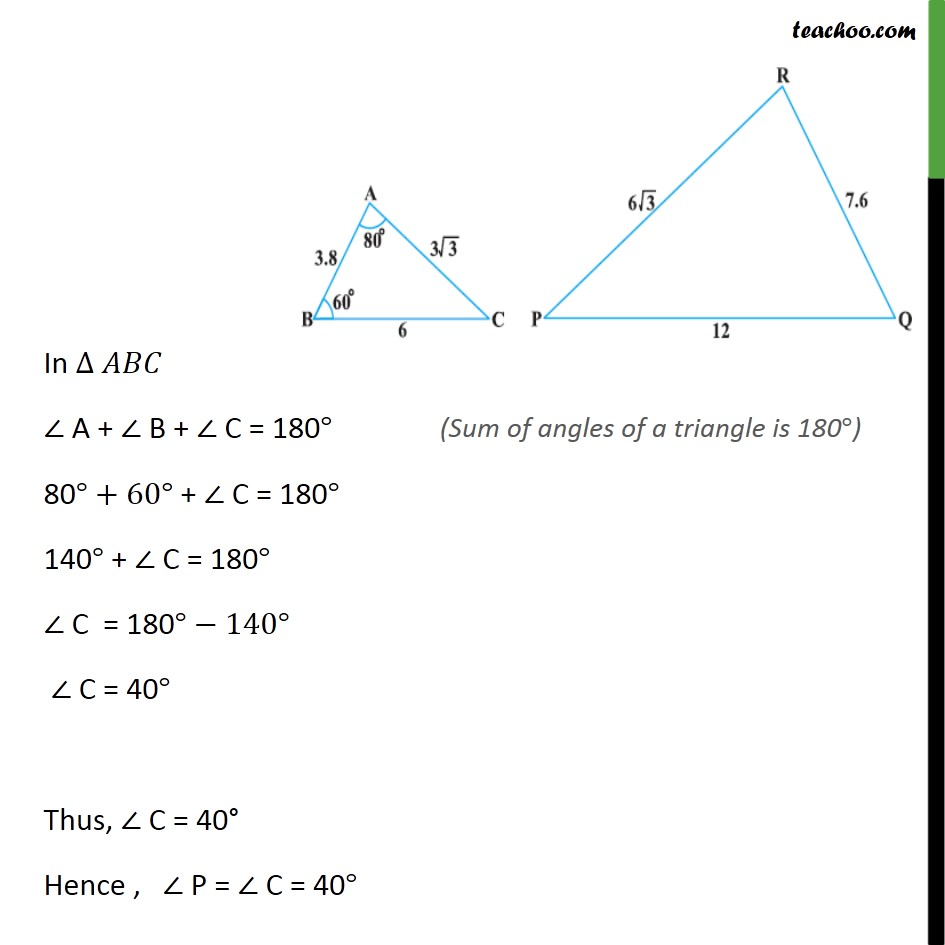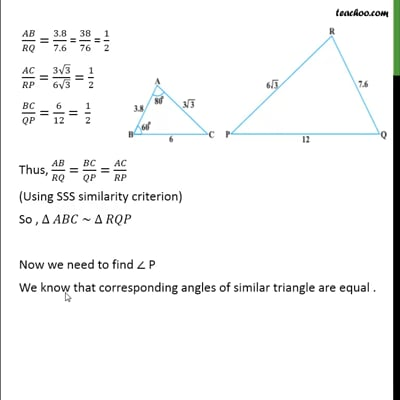Chapter 6 Class 10 Triangles

Class 10
Important Questions for Exam - Class 10This video is only available for Teachoo black users

Solve all your doubts with Teachoo Black (new monthly pack available now!)

### Transcript

Example 5 Observe figure and then find P. Given: AB = 3.8 , PQ = 6 3 , BC = 6 , QR = 12 , AC = 3 3 , PR = 7.6 To find:- angle P i.e. P Solution:- in Let us find the ratio of sides / =3.8/7.6 = 38/76 = 1/2 / =(3 3)/(6 3)=1/2 / =6/12= 1/2 Thus, / = / = / (Using SSS similarity criterion) So , ~ Now we need to find P We know that corresponding angles of similar triangle are equal . Therefore, C = P In A + B + C = 180 80 +60 + C = 180 140 + C = 180 C = 180 140 C = 40 Thus, C = 40 Hence , P = C = 40